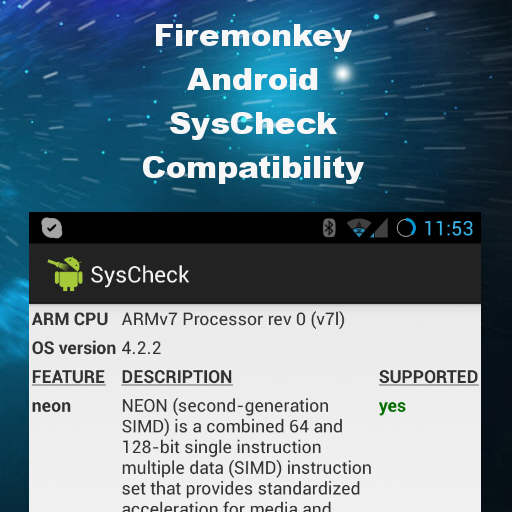# Draw Cos and Sin On A TPlotGrid In Delphi XE6 Firemonkey On Android And IOSEmbarcadero has a sample that comes with Delphi XE6 Firemonkey which demonstrates plotting lines on a grid using the TPlotGrid component. The TPlotGrid component is basically a grid component where you can draw things like lines or charts and have them match up with the grid background of the component. This component works on Android, IOS, Windows, and OSX. In the sample simple Sin and Cos lines are plotted into a TPolygon and then drawn on the plot grid in the OnPaint event. It has two functions CalculateCos and CalculateSin where the TPolygon is filled up and then DrawPolygon is used to draw it out. There is a function called SetParams where the resolution, radian, origin, and interval of the points is calculated based on the size of the component. Resolution is the precision of the graph. Radian is the angle. Origin is the middle point of the TPlotGrid. And interval is the space between two points. Here is the SetParams function from the sample: